Mobile QR Code1. (Department of Electronic Engineering, Sogang University, Seoul 04107, Korea)
2. (Samsung Electronics Company, Limited, Hwaseong 18448, Korea)

Analog-to-digital converter (ADC), successive-approximation register (SAR), capacitor calibration, low-noise comparator

## I. INTRODUCTION

Due to advances in process technologies, low-power successive-approximation register (SAR) analog-to-digital converters (ADCs) primarily based on digital circuits are increasingly used in high-resolution applications where pipeline and oversampling structures have been commonly employed (1-4). However, in SAR ADCs, when the number of processing bits increases, the required number of unit capacitors likewise increases exponentially, thus lowering area efficiency. To overcome this disadvantage, there have been active efforts to develop a variety of custom capacitors smaller than the minimum capacitor provided in the process. However, it is difficult to guarantee the matching accuracies of unit capacitors, leading to a deterioration in linearity.

Capacitor trimming is widely used as an intuitive and effective method to ensure high linearity. In the case of previously reported trimming methods, (5-9) require a separate calibration digital-to-analog converter (DAC) or physical trim capacitors to compensate the nonlinearity of the DAC, and (10) requires a separate resistive DAC. There have been studies on various capacitor calibration schemes to secure the linearity of high-resolution SAR ADCs. However, such methods have been limited by various obstacles: need for an additional calibration DAC with custom calibration capacitor arrays smaller than the unit capacitor, or a complicated calculation that makes system application difficult. In addition, if it is difficult to secure a separate calibration cycle in an application system, a high-resolution specification may not be achievable (11-13).

Meanwhile, even if the capacitor array secures linearity, when limited by a low supply voltage and small input signal range, the comparator noise becomes one of the major restricting components in the performance of the SAR ADC. Methods such as majority voting that averages the noise with additional conversion cycles have been suggested to overcome these limitations (14-17).

This work proposes a calibrated 14-bit 10 MS/s 28 nm CMOS SAR ADC which reuses segmented reference voltages. In the proposed calibration scheme, there is no need for extra calibration DACs or custom calibration capacitors that are commonly smaller than the unit capacitor, thus reducing the complexity of the calibration circuit. The comparator with a noise-reduction capacitor achieves low-noise performance with low-power.

The rest of this paper is organized as follows. Section II discusses the overall architecture and calibration scheme of the proposed ADC. The detailed circuit techniques are illustrated in Section III. In Section IV, the measured results of the prototype ADC are summarized. Finally, conclusions are drawn in Section V.

## II. Proposed ADC and Calibration

### 1. Proposed SAR ADC Architecture

The overall structure of the proposed 14-bit 10 MS/s 28 nm CMOS SAR ADC is shown in Fig. 1. It is composed of a capacitor-resistor (C-R) hybrid DAC with a calibration scheme, a comparator, a SAR logic, a calibration logic, and control and timing circuits. The proposed C-R hybrid DAC with common-mode voltage (VCM)-based switching determines the upper 9 bits using the binary-weighted capacitor array. The remaining lower 5 bits are determined by using the unit capacitors and the reusable segmented reference voltages divided from a simple binary-weighted resistor string (18,19). Since only 520 unit capacitors are used to implement a 14-bit DAC, the proposed DAC structure can reduce the number of unit capacitors required.

In the C-R hybrid DAC, the unit capacitance is determined to be 10fF based on the input signal range of 2.0V$_{\mathrm{P-P}}$, kT/C noise, and capacitor matching characteristics (1,2). The resistor string used to determine the lower 5 bits has a binary-weighting structure. The capacitor calibration is applied to the uppermost 4 capacitors -128$C_{\mathrm{U}}$, 64$C_{\mathrm{U}}$, 3$2C_{\mathrm{U}}$, and 16$C_{\mathrm{U}}$ -which have the biggest impact on the linearity of the capacitor array, minimizing performance degradation that may result from capacitor mismatch. The proposed calibration scheme, instead of using an additional calibration DAC or a custom calibration capacitor smaller than the unit capacitor, reuses the segmented reference voltages, reducing the complexity of the calibration circuit. The comparator with noise-reduction capacitors is employed not only to determine the 14-bit digital code during SAR operation but also to measure the abnormality of the capacitor array during calibration.

Fig. 3. Implementation example of the proposed capacitor calibration.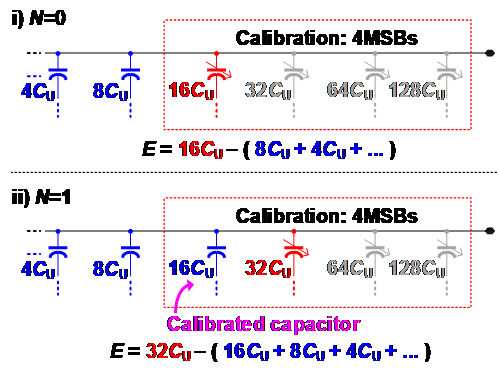The resistor string used to determine the lower 5 bits has a binary-weighting structure. The segmented reference voltages are not highly accurate due to mismatches between resistors. These non-ideal reference segment voltages can affect the linearity of the C-R hybrid DAC. However, the non-ideal components of the resistor string are attenuated at the DAC output by a ratio of the unit capacitance to the total capacitance of the DAC. As a result, the required matching accuracy of the resistor string is reduced to approximately 7 bits (19). Meanwhile, since the resistance of the R-DAC determines the current between VREF+ and VREF-, it affects the settling behavior of the reference voltages. Given the required accuracy and the settling time of the segmented reference voltages, a unit resistance of 400Ω is used. Based on Monte Carlo simulation, the estimated matching accuracy of the designed resistor string for the given process satisfies over 7 bits, which meets the required matching accuracy sufficiently. In addition, since the noise of the resistor string is also attenuated at the DAC output, it hardly contributes to the performance degradation of the entire C-R hybrid DAC.

The glitches in the reference voltages due to R-DAC switching kickback noise occur periodically. During the lower 5-bit conversion cycles using R-DAC, the reference voltages are only connected to the 10fF unit capacitor through the resistor ladder, so the glitch size of the reference voltages are small and the reference voltages stabilize within a given time.

### 2. Proposed Capacitor Calibration Scheme

The flow chart of the capacitor calibration algorithm is shown in Fig. 2. The proposed capacitor calibration scheme is applied to the most significant 4-bit capacitors. The calibration target capacitors are assigned in order from the fourth uppermost capacitor, 16$C_{\mathrm{U}}$, where N is 0, to the first uppermost capacitor, 128$C_{\mathrm{U}}$, where N is 3. The objective for calibration is to transform the DAC into an ideal binary-weighted capacitor array. The difference, E, between the capacitance of the calibration target capacitor, $2^{N+4}$$C_{\mathrm{U}}, and the total summed capacitance of the lower capacitors, \sum _{\mathrm{k}=0}^{3- \mathrm{N}}2^{\mathrm{k}}C_{\mathrm{U}} + C_{\mathrm{U}}, is compared. Depending on the comparison result, the value of 2^{N+4}$$C_{\mathrm{U}}$ is adjusted to be bigger or smaller. The implementation example, where N is 0 or 1, is shown in Fig. 3, respectively.

Fig. 4 shows the procedure for the proposed capacitor calibration in a simplified single-ended version where the calibration target capacitor is 16$C_{\mathrm{U}}$. As shown in Fig. 4(a), during the reference sampling phase, 16$C_{\mathrm{U}}$ and the lower capacitors sample the complementary reference voltages each other, and all upper capacitors than 16$C_{\mathrm{U}}$ are reset. Then, the capacitance of the calibration target capacitor and the total summed capacitance of the lower capacitors are compared during the comparator decision phase. Depending on the comparison result, the capacitance of the calibration target capacitor is adjusted using the combination of external digital codes, $D_{\mathrm{X}}$<2:0>, as shown in Fig. 4(b).

Fig. 4. Capacitor calibration procedure (a) reference sampling, comparator decision, (b) variable capacitor adjustment.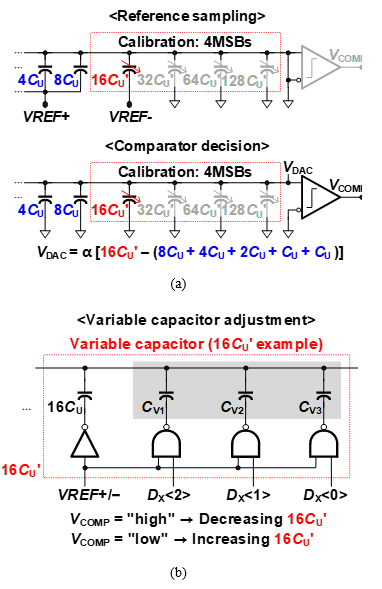Fig. 5. Conventional calibration circuit based on custom-built capacitors.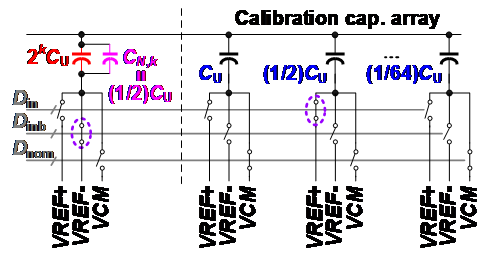Fig. 6. Proposed calibration circuit based on reusable segmented reference voltages.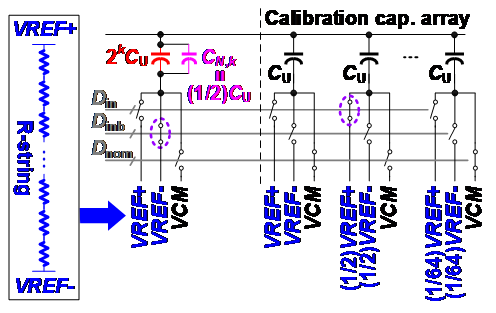## III. Circuit Implementation

### 1. Calibration Reusing Segmented Reference Voltages

Fig. 5 and 6 show the calibration circuit based on a capacitor array. When the calibration target is the k-th weighted capacitor, assume that $C_{\mathrm{N,k}}$ = (1/2)·$C_{\mathrm{U}}$ is a parasitic component of the ideal capacitance, $2^{\mathrm{k}}$$C_{\mathrm{U}}$, and the target capacitor is connected to the reference voltage VREF-. In the conventional capacitor calibration scheme, as shown in Fig. 5, the complementary reference voltage, VREF+, will be connected to the additional custom-built capacitor (1/2)·$C_{\mathrm{U}}$ in the calibration capacitor array to compensate for the non-ideal value of the calibration target capacitor.

In the proposed calibration scheme, as shown in Fig. 6, the non-ideal value can be compensated for by connecting (1/2)·VREF+ to the unit capacitor in the calibration capacitor array. The proposed calibration circuit in this work does not need extra custom-built capacitors and the calibration capacitor array can be laid out together with the sampling capacitor array, reducing the required chip area and the circuit complexity. The expected maximum mismatch range is determined based on the Monte Carlo simulated maximum capacitor mismatch range, the parasitic components between the adjacent capacitors, and the design margin.

### 2. Low-noise Comparator with a Noise-reduction Capacitor

Fig. 7. Low-power low-noise comparator with noise-reduction capacitor.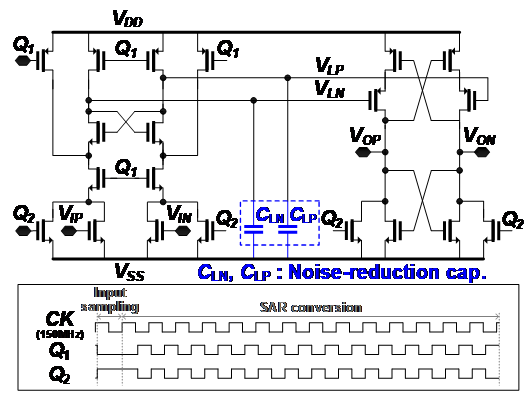Fig. 8. Simulated power consumption and noise performance of the comparator corresponding to noise-reduction capacitor.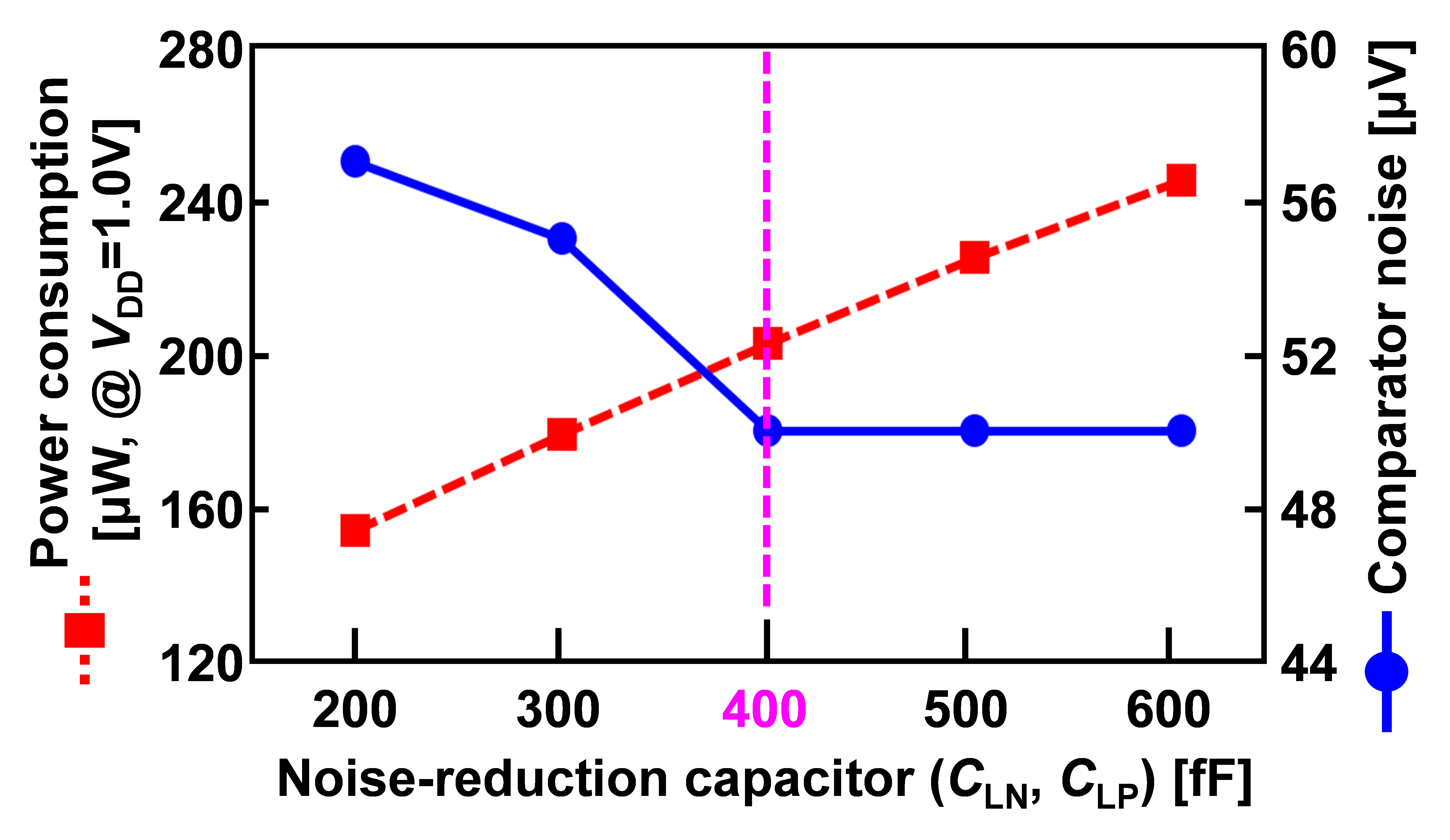Fig. 10. (a) Power distribution, (b) noise contribution of the prototype ADC.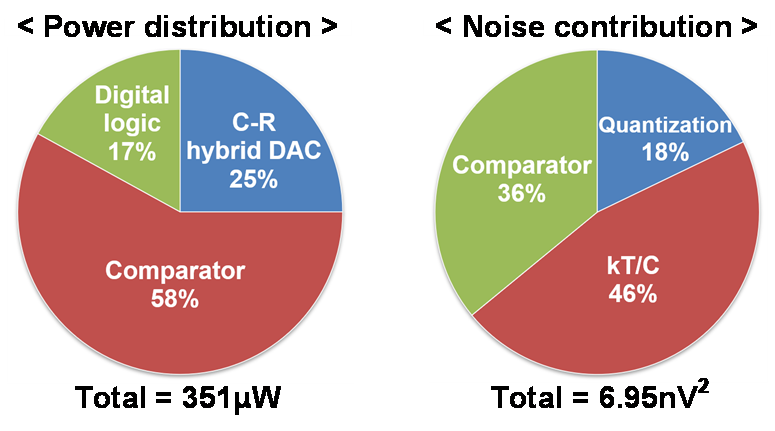To apply the proposed calibration scheme, it is necessary to have a process to compare the capacitance of the calibration target capacitor and the sum of the lower capacitors. For this process, a low-noise comparator with 14-bit accuracy is required. As shown in Fig. 7, the proposed SAR ADC employs a noise-reduction capacitor-based comparator. The proposed comparator attenuates noise using the noise-reduction capacitors, $C_{\mathrm{LP}}$ and $C_{\mathrm{LN}}$, connected to the first-stage latch output terminals (20). The simulated noise performance and power consumption of the proposed comparator corresponding to the noise-reduction capacitor is shown in Fig. 8. In the proposed comparator, given the required noise performance, power consumption, and chip area, both $C_{\mathrm{LP}}$ and $C_{\mathrm{LN}}$ are selected to be 400 fF.

## IV. Measurement Results

The prototype 14-bit 10 MS/s SAR ADC reusing segmented reference voltages is fabricated in a 28 nm CMOS process. Fig. 9 shows the layout and die chip photo with an active die area of 0.062 mm$^{2}$. Fig. 10(a) shows the power distribution of the ADC. It consumes 351 μW at 10 MS/s with a 1.0 V supply voltage. The power consumption of the reference buffer and digital output buffer is not included. The comparator consumes the most power (58%) to achieve target speed. Fig. 10(b) shows the simulated noise contribution of the ADC. The kT/C noise occupies the highest proportion (46%) of the total noise.

Fig. 11 shows the test board and measurement setup. The DC bias voltages are provided by the power supply. A fully differential sinusoidal input signal and a clock signal are applied using signal generators. The output data is collected by the logic analyzer and analyzed using MATLAB.

Fig. 12. Measured FFT spectrum of the prototype ADC ($f_{\mathrm{IN}}$ = 1.1 MHz).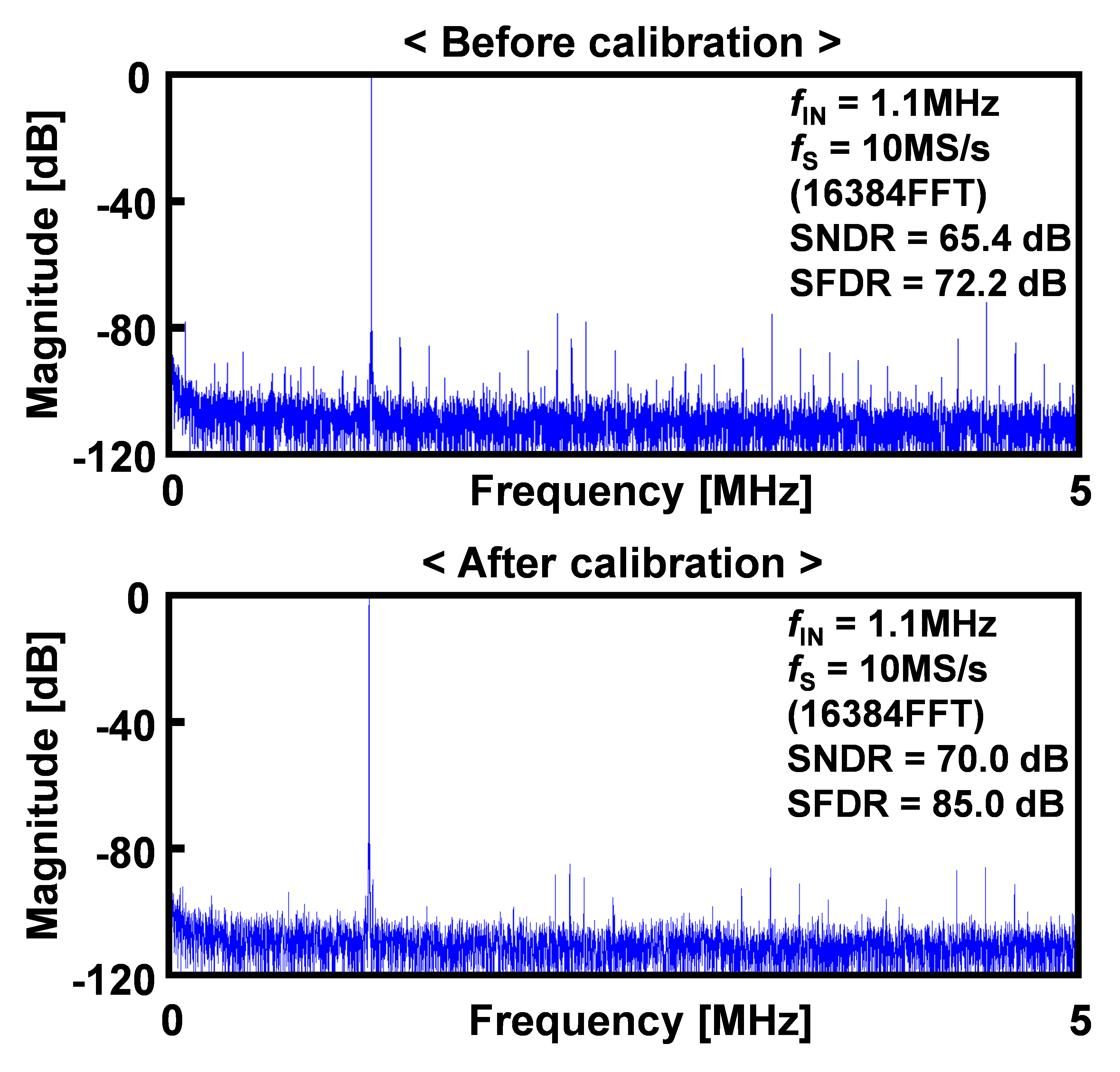Fig. 13. Measured FFT spectrum of the prototype ADC ($f_{\mathrm{IN}}$ = 4.8 MHz).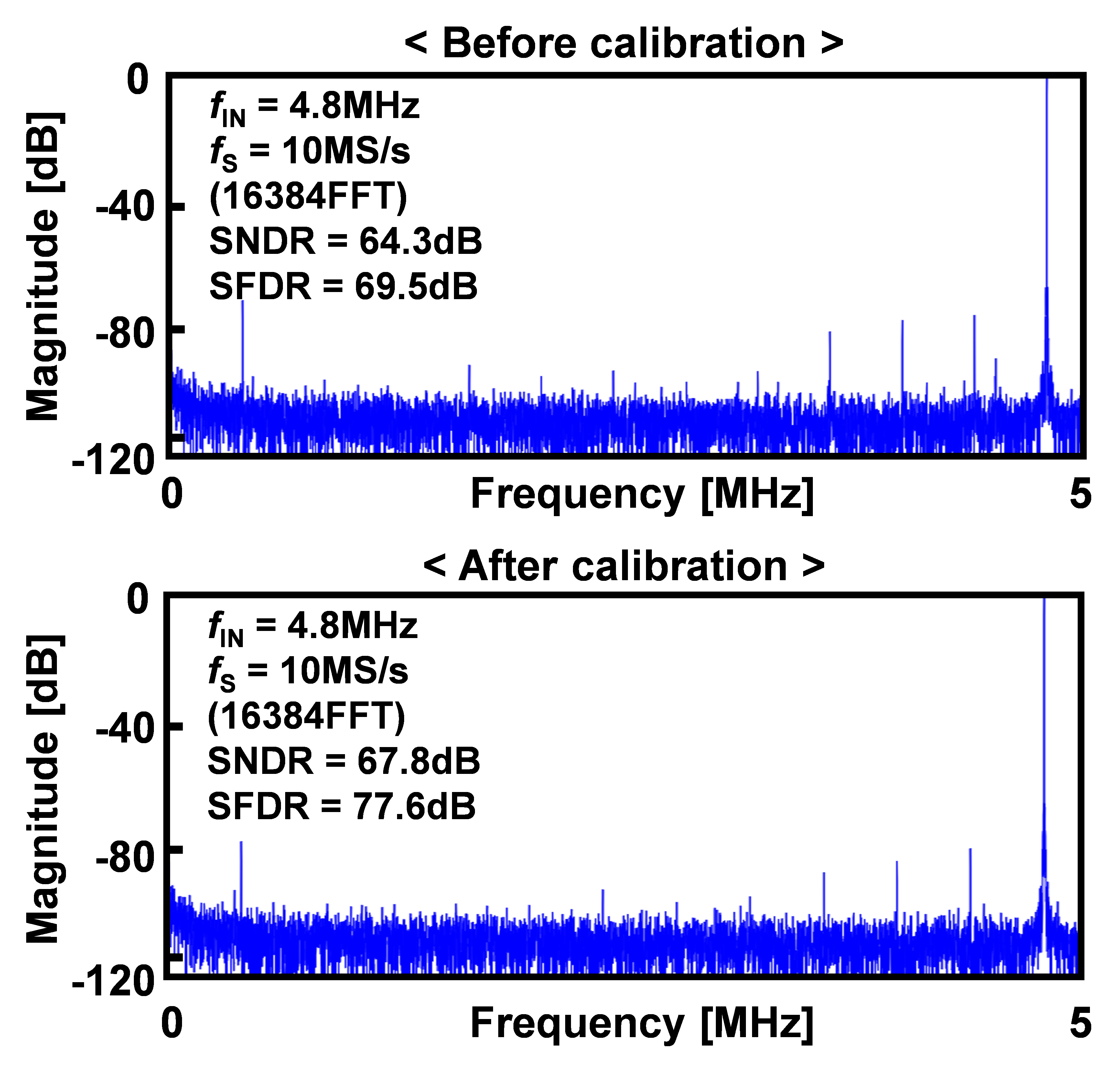Before calibration, the measured differential non-linearity (DNL) and integral non-linearity (INL) are within 1.87 LSB and 3.95 LSB, respectively, and after calibration, the measured DNL and INL are within 1.59 LSB and 2.92 LSB, respectively, as shown in Fig. 11.

The measured FFT spectrum at 10 MS/s with a 1.1 MHz sinusoidal input is shown in Fig. 12. Before calibration, the maximum signal-to-noise-and-distortion ratio (SNDR) and spurious-free dynamic range (SFDR) are 65.4 dB and 72.2 dB, respectively. They are improved to 70.0 dB and 85.0 dB, respectively, with the proposed calibration.

The measured FFT spectrum at 10 MS/s with a Nyquist input is shown in Fig. 13. Before calibration, the maximum SNDR and SFDR are 64.3 dB and 69.5 dB, respectively. After calibration, the maximum SNDR and SFDR are 67.8 dB and 77.6 dB, respectively.

As illustrated in Fig. 14(a), the SNDR and SFDR are measured with sampling rates ranging from 2 MS/s to 10 MS/s for a 1.1 MHz sinusoidal input signal. When the sampling rate increases to 10 MS/s, the SNDR and SFDR are maintained above 65.1 dB and 70.8 dB, respectively, before calibration; they are maintained above 69.9 dB and 83.9 dB, respectively, after calibration. Fig. 14(b) shows the measured SNDR and SFDR with increasing input frequencies at an operating speed of 10 MS/s. As the input frequency increases to the Nyquist frequency, the SNDR and SFDR are maintained above 64.3 dB and 69.5 dB, respectively, before calibration; they remain above 67.8 dB and 77.6 dB, respectively, after calibration.

Fig. 14. Measured SNDR and SFDR performance of the prototype ADC versus (a) $f_{\mathrm{S}}$, (b) $f_{\mathrm{IN}}$.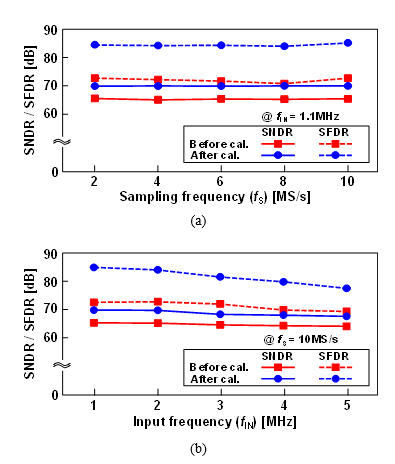Table 1. Performance summary and comparison of recently reported ADCs

 This work JSSC’17 (21) JSSC’19 (22) CICC’17 (23) TCASII’17 (24) JSSC’19 (25) JSSC’19 (26) Resolution [bits] 14 13 13 14 14 14 16 Speed [MS/s] 10 6.4 10 2 30 75 24 Supply [V] 1.0 1.0 0.7 1.6-3.6 1.2/2.5 1.2/1.8 1.1 Input range [VP-P] 2.0 2.0 1.4 3-6 N/A 1.2 2.2 Calibration Before After After X X After After X DNL [LSB] -0.84 / +1.87 -0.82 / +1.59 <1.08 -0.78 / 0.65 -0.88 / +0.95 N/A -0.42 / +0.41 -0.70 +0.60 INL [LSB] -3.95 / +3.56 -2.88 / +2.92 <3.79 -2.38 / +2.51 -2.00 / +1.98 N/A -0.53 / +0.56 -8.30 / +6.60 SNDR [dB] 65.4 70.0 64.06 67.6 77.76 77.1 77.8 75.3 SFDR [dB] 72.2 85.0 81.88 77.2 89.7 97.5 92.1 89.4 Power [mW] 0.351 0.046 0.0625 0.85 38.0 24.9 5.0 *FoMW [fJ/conv.] 13.6 (@LF) 17.5 (@Nyquist) 5.5 3.2 67.0 217 52.3 44.7 **FoMS [dB] 171.5 (@LF) 169.3 (@Nyquist) 172.5 176.6 168.5 163.1 172.6 169.0 Process [CMOS] 28 nm 40 nm 40 nm 65 nm 40 nm 65 nm 90 nm Area [mm2] 0.062 0.0675 0.013 0.085 0.236 0.342 0.368 *FoMW = Power/(2ENOB·2·BW) **FoMS = DR + 10·log10(BW/Power)

After calibration, the performance improvement is more limited than expected. The statistical analysis process to reflect the capacitor trimming by analyzing the capacitor mismatch error is insufficient. In particular, the trimming resolution of the calibration is low, which limits the improvement of ADC performance. By increasing the trimming resolution of the correction and supplementing the statistical algorithm, the expected target performance can be obtained in the proposed structure.

The performance of the prototype ADC is summarized and compared with previous calibrated 13- to 14-bit ADCs in Table 1.

## V. CONCLUSIONS

This paper presents a calibrated 14-bit 10 MS/s 28 nm CMOS SAR ADC. The proposed ADC employs a C-R hybrid DAC with only 520 unit capacitors, a simple 5-bit resistor string for 14 bits and a noise-reduction capacitor-based comparator, while improving linearity with the proposed calibration scheme that reuse segmented reference voltages from the resistor string. With the proposed calibration, the prototype ADC achieves a measured DNL and INL within 1.59 LSB and 2.92 LSB, respectively, with a maximum SNDR and SFDR of 70.0 dB and 85.0 dB at 10 MS/s, respectively, consuming 351 μW at a 1.0 V supply voltage.

### ACKNOWLEDGMENTS

This work was supported by Samsung Electronics Co., Ltd (IO201210-08008-01), the IDEC of KAIST, and the MSIT under the ITRC program (IITP-2021-2018-0-01421) supervised by the IITP.

### REFERENCES

1
Hurrell C. P., Lyden C., Laing D., Hummerston D., Vickery M., Dec 2010, An 18 b 12.5 MS/s ADC with 93 dB SNR, IEEE J. Solid-State Circuits, Vol. 45, No. 12, pp. 2647-26542
Shen J., Apr 2018, A 16-bit 16-MS/s SAR ADC with on-chip calibration in 55-nm CMOS, IEEE J. Solid-State Circuits, Vol. 53, No. 4, pp. 1149-11603
Hsu C.-W., Mar 2018, A 12-b 40-MS/s calibration-free SAR ADC, IEEE Trans. Circuits Syst. I Reg. Papers, Vol. 65, No. 3, pp. 881-8904
Asghar S., Nov 2018, A 2-MS/s, 11.22 ENOB, extended input range SAR ADC with improved DNL and offset calculation, IEEE Trans. Circuits Syst. I Reg. Papers, Vol. 65, No. 11, pp. 3628-36385
Tan K. S., Aug 16 1983, On board self-calibration of analog-to-digital and digital-to-analog converters, U.S. Patent 4 399 4266
Yoshioka M., , A 10-b 50-MS/s 820-μW SAR ADC with on-chip digital calibration, in Proc. IEEE Int. Solid-State Circuits Conf, pp. 384-3857
Timko M. P., 1997, A/D converter with charge redistribution DAC and split summation of main and correcting DAC outputs, U.S. Patent 5 684 4878
Welland D. R., Callahan M. J., Nov 24 1987, Self-calibration method for capacitors in a monolithic integrated circuit, U.S. Patent 4 709 2259
Chen H. F., Jan 30 2007, Self-calibration circuit for capacitance mismatch, U.S. Patent 7 170 43910
Troster G., Herbst D., Jun 1988, Error cancellation technique for capacitor arrays in A/D and D/A converters, IEEE Trans. Circuits Syst., Vol. 35, No. 6, pp. 749-75111
Liu W., Feb 2009, A 600 MS/s 30 mW 0.13${\mathrm{\mu}}$m CMOS ADC array achieving over 60 dB SFDR with adaptive digital equalization, in IEEE ISSCC Dig. Tech. Papers, pp. 82-8312
McNeill J. A., Chan K. Y., Coln M. C. W., David C. L., Brenneman C., Oct 2011, All-digital background calibration of a successive approximation ADC using the, IEEE Trans. Circuits Sys. I Reg. Papers, Vol. 58, No. 10, pp. 2355-236513
Um J.-Y., Kim Y.-J., Song E.-W., Sim J.-Y., Park H.-J., Nov 2013, A digital-domain calibration of split-capacitor DAC for a differential SAR ADC without additional analog circuits, IEEE Trans. Circuits Sys. I Reg. Papers, Vol. 60, No. 11, pp. 2845-285614
Harpe P., Cantatore E., van Roermund A., Dec 2013, A 10b/12b 40 kS/s SAR ADC with data-driven noise reduction achieving up to 10.1b ENOB at 2.2 fJ/conversion-step, IEEE J. Solid-State Circuits, Vol. 48, No. 12, pp. 3011-301815
Namgoong M. Ahmadi and W., Sep 2013, A 3.3 fJ/conversion-step 250 kS/s 10 b SAR ADC using optimized vote allocation, in Proc. IEEE Custom Integr. Circuits Conf. (CICC), Vol. 22, No. 25, pp. 1-416
Chen L., Sep 2015, A 10.5-b ENOB 645 nW 100kS/s SAR ADC with statistical estimation based noise reduction, in Proc. Custom Integr. Circuit Conf. (CICC), pp. 1-417
Fateh S., Nov 2015, A reconfigurable 5-to-14 bit SAR ADC for battery-powered medical instrumentation, IEEE Trans. Circuits Syst. I Reg. Papers, Vol. 62, No. 11, pp. 2685-269418
Zhu Y., Jun 2010, A 10-bit 100-MS/s reference-free SAR ADC in 90nm CMOS, IEEE J. Solid-State Circuits, Vol. 45, No. 6, pp. 1111-112119
Park J.-S., Feb 2020, 12 b 50 MS/s 0.18 μm CMOS SAR ADC based on highly linear C-R hybrid DAC, IET Electronics Letters, Vol. 56, No. 3, pp. 119-12120
Xu H., Abidi A. A., Aug 2019, Analysis and design of regenerative comparators for low offset and noise, IEEE Trans. Circuits Syst. I Reg. Papers, Vol. 66, No. 8, pp. 2817-283021
Ding M., Feb 2017, A 46 μW 13 b 6.4 MS/s SAR ADC with background mismatch and offset calibration, IEEE J. Solid-State Circuits, Vol. 52, No. 2, pp. 423-43222
Chang K-.H., Hsieh C.-C., Oct 2019, A calibration-free 13-bit 10-MS/s full-analog SAR ADC with continuous-time feedforward cascaded op-amps, IEEE J. Solid-State Circuits, Vol. 54, No. 10, pp. 2691-270223
Park J., Nagaraj K., Ash M., Kumar A., A 12-/14-bit, 4/2MSPS, 0.085mm2 SAR ADC in 65nm using novel residue boosting, in Proc. Custom Integr. Circuit Conf. (CICC), pp. 1-424
Kr M., "Amer , Janssen E., Doris K., Murmann and B., Feb 2017, A 14-bit 30-MS/s 38-mW SAR ADC using noise filter gear shifting, IEEE Trans. Circuits Syst. II Exp. Briefs, Vol. 64, No. 2, pp. 116-12025
Xu H., Feb 2019, A 78.5-dB SNDR radiation- and metastability-tolerant two-step split SAR ADC operating up to 75 MS/s with 24.9-mW power consumption in 65-nm CMOS, IEEE J. Solid-State Circuits, Vol. 54, No. 2, pp. 441-45126
Hung T.-C., Kuo T.-H., May 2019, A 75.3-dB SNDR 24-MS/s ring amplifier-based pipelined ADC using averaging correlated level shifting and reference swapping for reducing errors from finite opamp gain and capacitor, IEEE J. Solid-State Circuits, Vol. 54, No. 5, pp. 1425-1435## Author

received the B.S. and M.S. degrees in electronic engi-neering from Sogang University, Seoul, Korea, in 2014 and 2016, respectively, where he is currently pursuing the Ph.D. degree. His research interests include high-speed and high-performance analog-to-digital converters. He was a recipient of a scholarship sponsored by Samsung electronics.

received the B.S. degree in electronic engineering from Sogang University, Seoul, Korea, in 2017, where he is currently pursuing the Ph.D. degree. His current research interests include analog and mixed-signal circuits, data converters, and sensor interfaces.

received the B.S. degree in electronic engineering from Sogang University, Seoul, Korea, in 2020, where he is currently pursuing the Ph.D. degree. His current interests are in the design of high-speed, high-resolution data converters, and high-speed mixed-mode integrated systems.

received the B.S., M.S., and Ph.D. degrees in electronic engineering from Sogang University, Seoul, Korea, in 2012, 2014, and 2020, respectively. He is currently with the Samsung Electronics Co., Ltd. and is developing high-speed data converter (ADC/DAC) circuits. His research interests include high-speed low-power data converter and mixed-signal circuits.

received the B.S. degree in electronic engineering from University of Seoul, Korea, in 2007, and the M.S. and Ph.D. degrees in electronic engineering from Sogang University, Korea, in 2013 and 2019, respectively. From 2007 to 2011, he was with Luxen Technologies, where he had developed various power-management and analog integrated circuits. Dr. An has been with the Samsung Electronics Co., Ltd. and is developing low-power high-speed high-resolution data converters.

received the B.S. and M.S. degrees from Sungkyunkwan University, Suwon, Korea, in 2014 and 2016, respectively. He joined Samsung Electronics, Hwaseong, South Korea, in 2016. Currently, he is an engineer in Samsung Foundry, where he is researching the high-speed CMOS data converters.

received the master’s and Ph.D. degrees from Sogang University, Seoul, South Korea, in 2003 and 2007, respectively. He joined Samsung Electronics, Hwaseong, South Korea, in 2010. Currently, he is a principle engineer in Samsung Foundry, leading data converter development. His major fields are high-speed data converters and application specific analog front-ends for various applications such as digital TV, 5G network, touch controller, CIS and automotive wired/wireless communications.

received the master’s and Ph.D. degrees from the University of California, Los Angeles, CA, USA, in 1998 and 2002, respectively. He joined Samsung Electronics, Hwaseong, South Korea, in 2006. Currently, he is a Master in Samsung Foundry, leading analog IP development. His expertise includes high-speed data converters and various analog front-ends for UHD digital TV, WiFi & 5G connectivity, automotive V2X, touch controller, and CMOS image sensor.

received the B.S. and M.S. degrees in electronic engi-neering from Sogang University, Seoul, Korea, in 1994 and 1996, respectively, and the Ph.D. degree in electrical engineering from Oregon State University, Corvallis, in 2005. From 1996 to 2001, he was a Design Engineer at Samsung Electronics, Kiheung, Korea, working on mixed analog-digital integrated circuits. From 2005 to 2008, he was with Broadcom Corporation, Irvine, CA, working on AFE for digital TV. Currently, he is a Professor in the Department of Electronic Engineering, Sogang University. His research interests include high-speed, high-resolution data converters and low-voltage, low-power mixed-signal circuits design.

received the B.S. and M.S. degrees in electronic engineering from Seoul National University, Korea, in 1984 and 1986, respectively, and the Ph.D. degree in electrical and computer engineering from the University of Illinois, Urbana-Champaign, in 1991. He was with Analog Devices Semiconductor, Wilmington, MA, from 1990 to 1993, as a Senior Design Engineer. Since 1993, he has been with the Department of Electronic Engineering, Sogang University, Seoul, where he is currently a Professor. His current research interests include design and testing of high-resolution high-speed CMOS data converters, CMOS communication circuits, integrated sensors, and mixed-mode integrated systems.